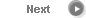#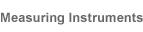# Precise Color Communication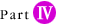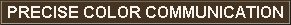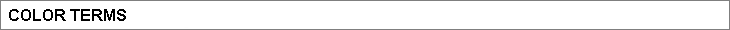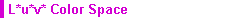The L*u*v* color space (also referred to as the CIELUV space) is one of the uniform color spaces defined by the CIE in 1976. The values of L*, u*, and v* are calculated according to the formulas below: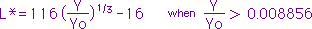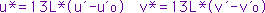where Y: Tristimulus value Y (tristimulus value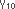can also be used.) u', v': Chromaticity coordinates from the CIE 1976 UCS diagram Yo, u'o, v'o: Tristimulus value Y (or) and chromaticity coordinates u', v' of the perfect reflecting diffuser.

 Color difference ∆E*uv in the L*u*v* color space, which indicates the degree of color difference but not the direction, is defined by the following equation: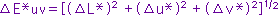where ∆L*, ∆u*, ∆v*: Difference in L*, u*, and v* values between the specimen color and the target color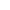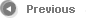7/12